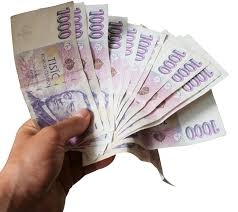# Eva and

Eva and Jana have a total of 560 CZK. Eva has a third more than Jana. How much CZK does each have?

A =  320 Kc
B =  240 Kc

### Step-by-step explanation:Did you find an error or inaccuracy? Feel free to write us. Thank you!

Tips for related online calculators
Need help calculating sum, simplifying, or multiplying fractions? Try our fraction calculator.
Do you have a system of equations and looking for calculator system of linear equations?